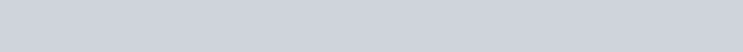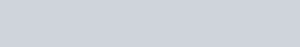# House Price prediction using Linear, Lasso and Ridge Regression in Python

In this tutorial, we will discuss about house price prediction in a major city like Banglore using Linear, Lasso and Ridge Regression with the help of Python programming.

You can know more details about Linear lasso and Ridge regression.

In the dataset, the customer will check whatever requirements they want for the dream house.

`So let’s try to implement the code`

Import the necessary Python libraries.

```import pandas as pd
import numpy as np
from sklearn.model_selection import train_test_split
from sklearn.linear_model import LinearRegression,Lasso,Ridge
from sklearn.preprocessing import OneHotEncoder,StandardScaler
from sklearn.compose import make_column_transformer
from sklearn.pipeline import make_pipeline
from sklearn.metrics import r2_score```

The first step is,

## Data Collection

`data=pd.read_csv("C:\\Users\\usersdrive\\OneDrive\\Desktop\\Bengaluru_House_Data.csv")`

To check whether the data set is loaded or not print the first five rows by using the head method.

`data.head()`

#### OUTPUT:-Now print the last five rows by using the tail method.

`data.tail()`

#### OUTPUT:-To check the dataset size.

`data.shape`

#### OUTPUT:-

`(13320, 9)`

Lets check the basic information about the dataset.

```data.info()
```

#### OUTPUT:-By seeing the above output there are only 3 columns in float.

To check the value counts

```for column in data.columns:
print(data[column].value_counts())
print("*",20)
```

#### OUTPUT:-To check wether any null value present in the given dataset.

`data.isnull().sum()`

#### OUTPUT:-There are 5502 null values present in the society column and 609 null values in the balcony column need to drop the columns otherwise the algorithm cant able to predict correctly.

To drop the null values

`data.drop(columns=['area_type','availability','society','balcony'],inplace=True)`

To check wether the columns are dropped or not.

`data.info()`

#### OUTPUT:-To fill the null values in one column can use the mean values and in one column use the standard deviation values.

To check mean and standard deviation values.

`data.describe()`

#### OUTPUT:-Now fill up the missing values one by one.

First try for the location.

`data['location'].value_counts()`

#### OUTPUT:-To fill the values in the sarjapur road.

`data['location']=data['location'].fillna('Sarjapur Road')`

To check the values.

`ata['size'].value_counts()`

#### OUTPUT:-Now fill the msiing values in the size with the 2 bhk.

`data['size']=data['size'].fillna('2 BHK')`

There are 73 missing values in the bath fill that values with the median value.

`data['bath']=data['bath'].fillna(data['bath'].median())`

To check wether the values is updated or not.

`data.info()`

#### OUTPUT:-`data['bhk']=data['size'].str.split().str.get(0).astype(int)`

Print the bhk greater than 20 these are the outliers of the data.

```#print the bhk greater than 20 these are outliers of data
data[data.bhk > 20]```

#### OUTPUT:-To check the square feet values.

`data['total_sqft'].unique()`

#### OUTPUT:-By seeing the above output we need to find the range for which the values are in hiphen need to add two and divide by two.

```def convertRange(x):
temp=x.split('-')
if len(temp)==2:
return(float(temp)+float(temp))/2
try:
return float(x)
except:
return None```
`data['total_sqft']=data['total_sqft'].apply(convertRange)`
`data.head()`

#### OUTPUT:-Now will make new column name Price per square feet it will helps for removing the outliers.

`data['price_per_sqft']=data['price']*100000/data['total_sqft']`
`data['price_per_sqft']`

#### OUTPUT:-`data.describe()`

#### OUTPUT:-The minimum price per square feet is 260 rs and the maximum price is 120 rs.

To check the location values.

`data['location'].value_counts()`

#### OUTPUT:-By seeing the above output there are total 1306 dummy values present in the data so need to reduce other wise it will not pass in to algorithm.

To reduce we will reduce the lambda function.

```data['location']=data['location'].apply(lambda x: x.strip())
location_count=data['location'].value_counts(```
`location_count`

#### OUTPUT:-Print if the location value is less than 10.

```location_count_less_10=location_count[location_count<=10]
location_count_less_10```

#### OUTPUT:-If location count less than 10 write other wise write back the location.

```data['location']=data['location'].apply(lambda x: 'other' if x in location_count_less_10 else x)
```
`data['location'].value_counts()`

#### OUTPUT:-### OUTLIER DETECTION AND REMOVAL

`data.describe()`

#### OUTPUT:-`(data['total_sqft']/data['bhk']).describe()`

#### OUTPUT:-If bhk greater than 300 need to keep that.

```data=data[((data['total_sqft']/data['bhk'])>=300)]
data.describe()
#minimum has changed```

#### OUTPUT:-To check the data shape.

`data.shape`

#### OUTPUT:-

`(12530, 7)`
`data.price_per_sqft.describe()`

#### OUTPUT:-To remove outliers need to introduce the function.

```def remove_outliers_sqft(df):
df_output=pd.DataFrame()
for key,subdf in df.groupby('location'):
m=np.mean(subdf.price_per_sqft)
st=np.std(subdf.price_per_sqft)
gen_df=subdf[(subdf.price_per_sqft > (m-st))&(subdf.price_per_sqft<=(m+st))]
df_output=pd.concat([df_output,gen_df],ignore_index=True)
return df_output
data=remove_outliers_sqft(data)
data.describe()
```

#### OUTPUT:-maximum value is reduced.

```def bhk_outlier_remover(df):
exclude_indices=np.array([])
for location,location_df in df.groupby('location'):
bhk_stats={}
for bhk,bhk_df in location_df.groupby('bhk'):
bhk_stats[bhk]={
'mean':np.mean(bhk_df.price_per_sqft),
'std':np.std(bhk_df.price_per_sqft),
'count':bhk_df.shape
}
for bhk,bhk_df in location_df.groupby('bhk'):
stats=bhk_stats.get(bhk-1)
if stats and stats['count']>5:
exclude_indices=np.append(exclude_indices,bhk_df[bhk_df.price_per_sqft<(stats['mean'])].index.values)
return df.drop(exclude_indices,axis='index')
```
`data=bhk_outlier_remover(data)`
`data.shape`

#### OUTPUT:-

`(7361, 7)`

This is the cleaned data.

`data`

#### OUTPUT:-Drop the size and price_per_sqft column.

`data.drop(columns=['size','price_per_sqft'],inplace=True)`

To check the cleaned data.

```#cleaned data
```

#### OUTPUT:-Send the cleaned data in to the csv file.

`data.to_csv('cleaned_data.csv')`

Divide the data in to the train and the test split.

```X=data.drop(columns=['price'])
y=data['price']```
`X_train,X_test,y_train,y_test=train_test_split(X,y,test_size=0.2,random_state=0)`

Print the split data.

```print(X_train.shape)
print(X_test.shape)```

#### OUTPUT:-

```(5888, 4)
(1473, 4)```

Applying the 3 types of the regression.

## LINEAR REGRESSION

`column_trans=make_column_transformer((OneHotEncoder(sparse=False),['location']),remainder='passthrough')`
`scaler=StandardScaler()`
`lr=LinearRegression(normalize=True)`
`pipe=make_pipeline(column_trans,scaler,lr)`
`pipe.fit(X_train,y_train)`

#### OUTPUT:-To check the r score value for the linear regression.

`y_pred_lr=pipe.predict(X_test)`
`r2_score(y_test,y_pred_lr)`

#### OUTPUT:-

`0.823438105512173`

Now same thing we will do with the lasso regression.

## LASSO REGRESSION

`lasso=Lasso()`
`pipe=make_pipeline(column_trans,scaler,lasso)`
`pipe.fit(X_train,y_train)`

#### OUTPUT:-To check the accuracy of the lasso regression.

```y_pred_lasso=pipe.predict(X_test)
r2_score(y_test,y_pred_lasso)```

#### OUTPUT:-

`0.8128285650772719`

The accuracy is nearly 81 it is good to apply.

Now apply the ridge regression.

## RIDGE REGRESSION

`ridge=Ridge()`
`pipe=make_pipeline(column_trans,scaler,ridge)`
`pipe.fit(X_train,y_train)`

#### OUTPUT:-To check the accuracy score.

```y_pred_ridge=pipe.predict(X_test)
r2_score(y_test,y_pred_ridge)```

#### OUTPUT:-

`0.8234146633312649`

Now lets compare the all three regression accuracy scores.

```print("No Regularization: ",r2_score(y_test,y_pred_lr
))
print("Lasso: ",r2_score(y_test,y_pred_lasso))
print("Ridge: ",r2_score(y_test,y_pred_ridge))```

#### OUTPUT:-Out of three ridge regression is best way to apply.